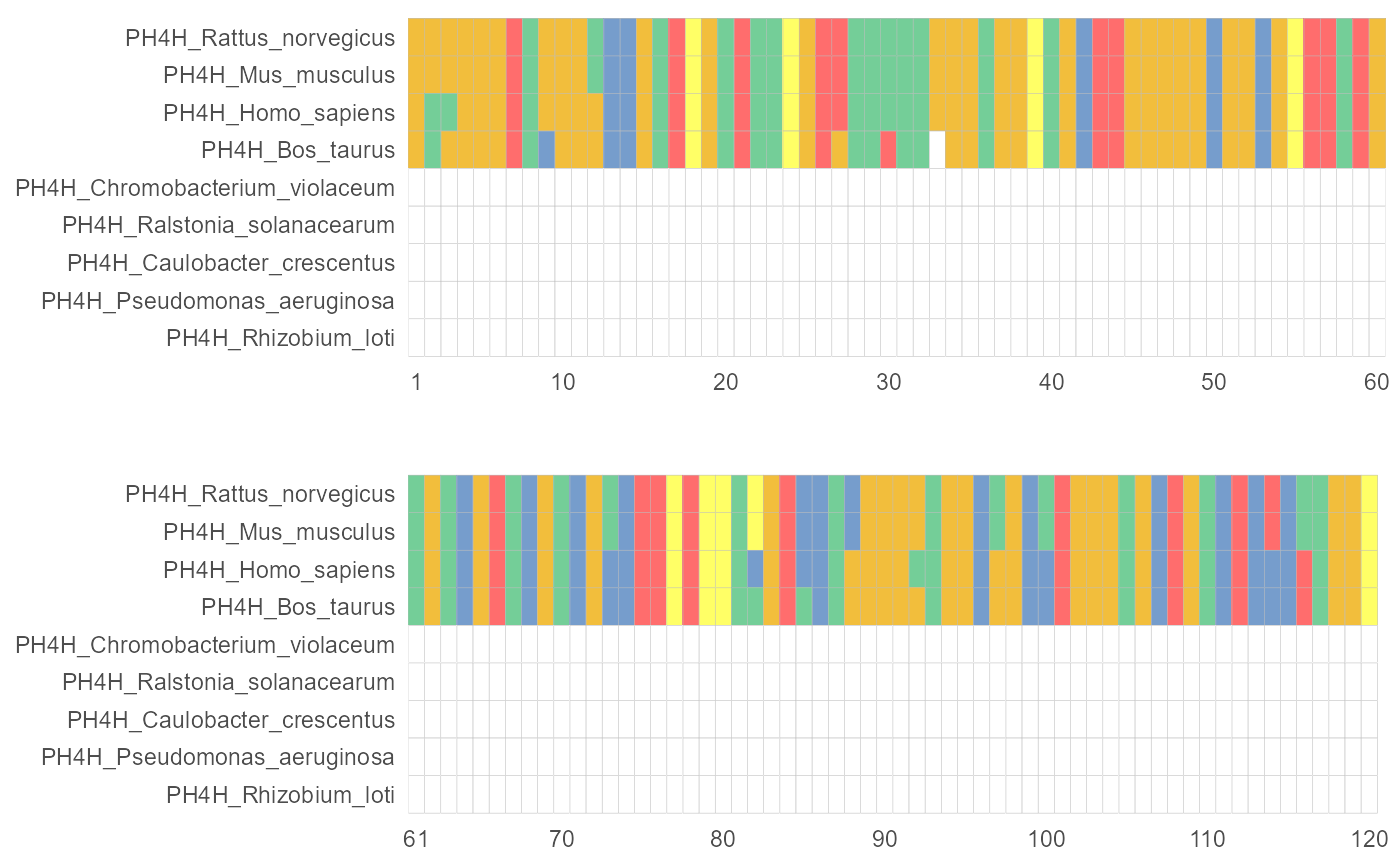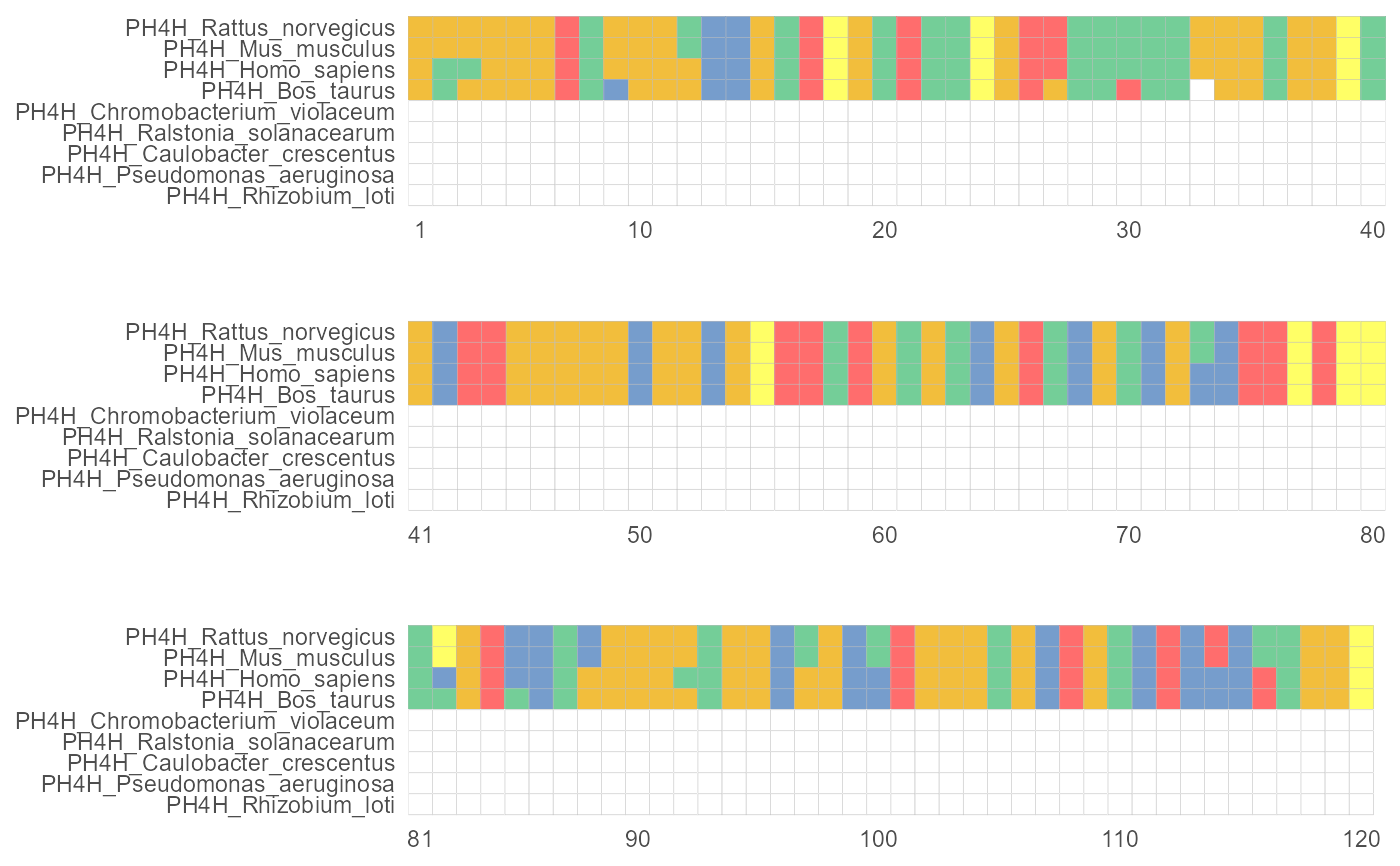The MSA would be plot in a field that you set.

facet_msa(field)

## Arguments

field a numeric vector of the field size.

ggplot layers

Lang Zhou

## Examples

library(ggplot2)
f <- system.file("extdata/sample.fasta", package="ggmsa")
# 2 fields
ggmsa(f, end = 120, font = NULL, color="Chemistry_AA") + facet_msa(field = 60)
#> Scale for 'x' is already present. Adding another scale for 'x', which will
#> replace the existing scale.#> Coordinate system already present. Adding new coordinate system, which will replace the existing one.# 3 fields
ggmsa(f, end = 120, font = NULL,  color="Chemistry_AA") + facet_msa(field = 40)
#> Scale for 'x' is already present. Adding another scale for 'x', which will
#> replace the existing scale.#> Coordinate system already present. Adding new coordinate system, which will replace the existing one.